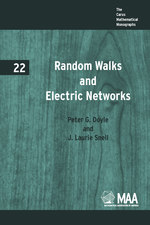# Random Walks and Electric Networks

PETER G. DOYLE
J. LAURIE SNELL
Volume: 22
Edition: 1
Pages: 174
https://www.jstor.org/stable/10.4169/j.ctt5hh804

1. Front Matter
(pp. i-vi)
2. PREFACE
(pp. vii-x)
(pp. xi-xiv)
4. ### Part I. Random Walks on Finite Networks

• CHAPTER 1 RANDOM WALKS IN ONE DIMENSION
(pp. 3-14)

A random walk, or drunkard’s walk, was one of the first chance processes studied in probability; this chance process continues to play an important role in probability theory and its applications. An example of a random walk may be described as follows:

A man walks along a 5-block stretch of Madison Avenue. He starts at cornerxand, with probability 1/2, walks one block to the right and, with probability 1/2, walks one block to the left; when he comes to the next corner he again randomly chooses his direction along Madison Avenue. He continues until he reaches corner 5,...

• CHAPTER 2 RANDOM WALKS IN TWO DIMENSIONS
(pp. 15-38)

We turn now to the more complicated problem of a random walk on a two-dimensional array. In Figure 2.1 we illustrate such a walk. The large dots represent boundary points; those markedEindicate escape routes and those markedPare police. We wish to find the probabilityp(x) that our walker, starting at an interior pointx, will reach an escape route before he reaches a policeman. The walker moves fromx= (a, b) to each of the four neighboring points$(a + 1,b),(a - 1,b),(a,b + 1),(a,b - 1)$with probability 1/4. If he reaches a boundary point, he remains at this point.

The corresponding...

• CHAPTER 3 RANDOM WALKS ON MORE GENERAL NETWORKS
(pp. 39-64)

Our networks so far have been very special networks with unit resistors. We will now introduce general resistor networks, and consider what it means to carry out a random walk on such a network.

A graph is a finite collection of points (also called vertices or nodes) with certain pairs of points connected by edges (also called branches). The graph is connected if it is possible to go between any two points by moving along the edges.

We assume thatGis a connected graph and assign to each edgexya resistanceRxy; an example is shown in Figure...

• CHAPTER 4 RAYLEIGH’S MONOTONICITY LAW
(pp. 65-82)

In this chapter we will study Rayleigh’s Monotonicity Law. This law from electric network theory will be an important tool in our future study of random walks. In this first section we will give an example of the use of this law.

Consider a random walk on streets of a city as in Figure 4.1. LetPescapebe the probability that a walker starting fromareachesbbefore returning toa.Assign to each edge a unit resistance and maintain a voltage of one volt betweenaandb;then a currentiawill flow into the circuit and...

5. ### Part II. Random Walks on Infinite Networks

• CHAPTER 5 PÓLYA’S RECURRENCE PROBLEM
(pp. 85-98)

In 1921 George Pólya  investigated random walks on certain infinite graphs, or as he called them, “street networks.” The graphs he considered, which we will refer to as lattices, are illustrated in Figure 5.1.

To construct ad-dimensional lattice, we take as vertices those points (x1, . . . ,xd) of Rdof whose coordinates are integers, and we join each vertex by an undirected line segment to each of its2dnearest neighbors. These connecting segments, which represent the edges of our graph, each have unit length and run parallel to one of the coordinate axes of...

• CHAPTER 6 RAYLEIGH’S SHORT-CUT METHOD
(pp. 99-118)

In its simplest form, Rayleigh’s method involves modifying the network whose resistance we are interested in so as to get a simpler network. We consider two kinds of modifications, shorting and cutting. Cutting involves nothing more than clipping some of the branches of the network, or what is the same, simply deleting them from the network. Shorting involves connecting a given set of nodes together with perfectly conducting wires, so that current can pass freely between them. In the resulting network, the nodes that were shorted together behave as if they were a single node.

The usefulness of these two...

• CHAPTER 7 THE CLASSICAL PROOFS OF PÓLYA’S THEOREM
(pp. 119-130)

In this chapter, all of our random walks will be simple. Letube the probability that a random walker, starting at the origin, will return to the origin. The probability that the walker will be there exactlyktimes (counting the initial time) is${u^{k - 1}}(1 - u)$· Thus, ifmis the expected number of times at the origin,

$m = \sum\limits_{k = 1}^\infty {k{u^{k - 1}}(1 - u)}$

$= \frac{1}{{1 - u}}$.

If$m = \infty$then$u=1$, and hence the walk is recurrent. If$m < \infty$then$u < 1$, so the walk is transient. Thusmdetermines the type of the walk.

We shall use an alternate expression form.Letunbe the probability...

• CHAPTER 8 RANDOM WALKS ON MORE GENERAL INFINITE NETWORKS
(pp. 131-150)

From now on we assume thatGis an infinite connected graph. We assume that it is of bounded degree, by which we mean that there is some integerEsuch that the number of edges from any point is at mostE.We assign to each edgexyofGa conductance${C_{xy}} > 0$with resistance${R_{xy}} = 1/{C_{xy}}$. The graphGtogether with the conductances$C = ({C_{xy}})$is called a network and denoted by$(G,{\rm{C}})$. Given a network$(G,{\rm{C}})$, we define a random walk by

${P_{xy}} = \frac{{{C_{xy}}}}{{{C_x}}}$

where${C_x} = {\sum _y}{C_{xy}}$. When all the conductances are equal, we obtain a random walk that moves along...

6. REFERENCES
(pp. 151-154)
7. INDEX
(pp. 155-159)